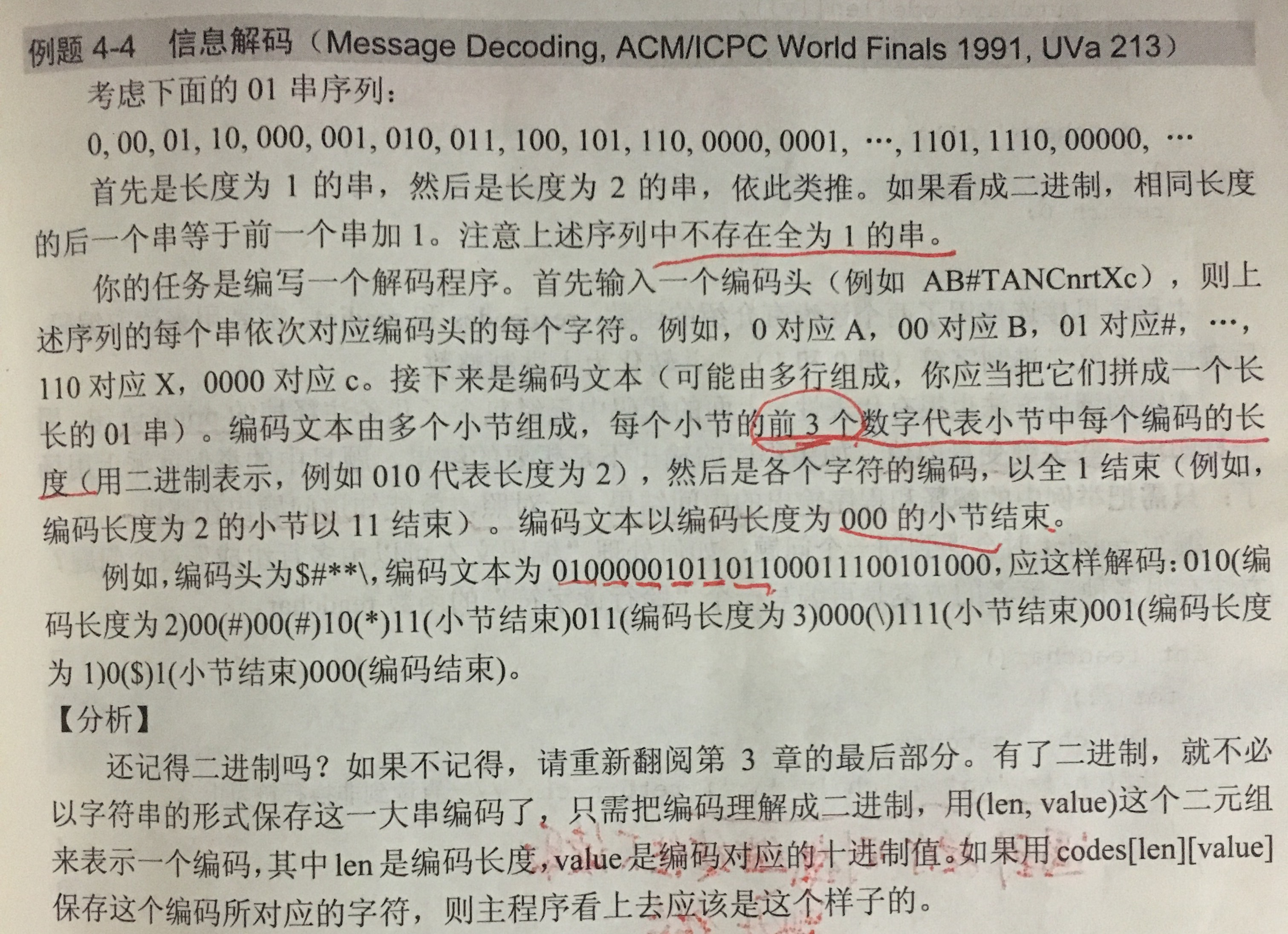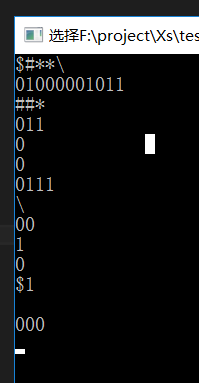# 信息解码（Message Decoding）ACM题目.....

1. 用 1<<n  表示 2^n

2.

codes[][]存储编码头；

Len表示  长度为Len的串

value表示  长度为Len的串的第value个值

3.

①先用循环读取 3位二进制数的 编码长度 （Len），并从二进制转换成十进制  (Len为0的时候结束循环)，

②然后往后每次读取Len位二进制数，并把Len位二进制数值转换成十进制value

③然后用 Lenvaluecodes[][] 里面找到对应的编码

④直到读取到 Len位二进制数全为1的时候，即 value = (1<<Len) -1 的时候 结束，跳到 ①

for(;;)循环调用getchar 读取字符，当遇到\n \t的时候，就跳过，然后往下读取，当读取到非换行符的时候，就跳出循环

#include<iostream>
using namespace std;

char codes[1 << 10 - 1];   // 即 codes[ 2^10 -1]

//读取字符
int readchar() {
for (;;) {
int ch = getchar();
if (ch != '\n'&&ch != '\t') //如果出现回车或换行 ，则跳过回车或换行，往后读取一个字符  （实现多行读取）
return ch;
}
}

//读取密文
int readint(int n) {
int i, v = 0;

for (i = n - 1; i >= 0; i--)
v += (readchar() - '0') * (1 << i); //转换成10进制
return v;
}

//读取编码头
int readcode() {
int len, i;
memset(codes, 0, sizeof(codes));

for (len = 1; len <= 9; len++) {
for (i = 0; i < (1 << len) - 1; i++) {
char ch = getchar();
if (ch == EOF)return 0;
if (ch == '\n' || ch == '\t')return 1; //遇到换行则读取结束
codes[len][i] = ch;
}
}
return 1; // 超过数codes组范围则读取结束
}

int main() {
int Len;
int p = 0, k = 0;
int value = 0;

readcode();
while (1) {
Len = 0; //编码长度

Len = readint(3);
if (Len == 0)break;

while (1) {
value = 0;

value = readint(Len); //读取Len位二进制数值，并转换成十进制

if (value == (1 << Len) - 1)break; //如果编码全为1，则跳出循环

putchar(codes[Len][value]);
}
putchar('\n');
}
return 0;
}